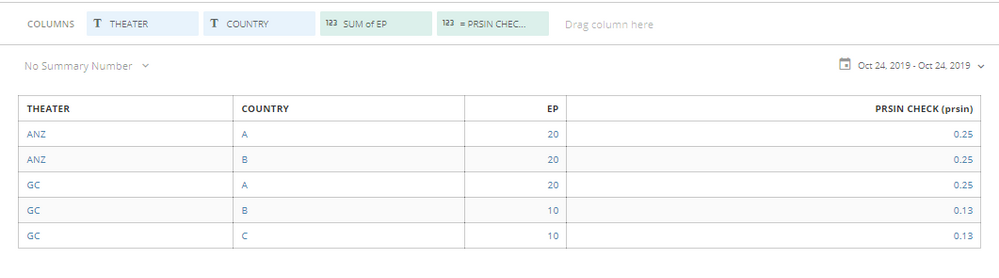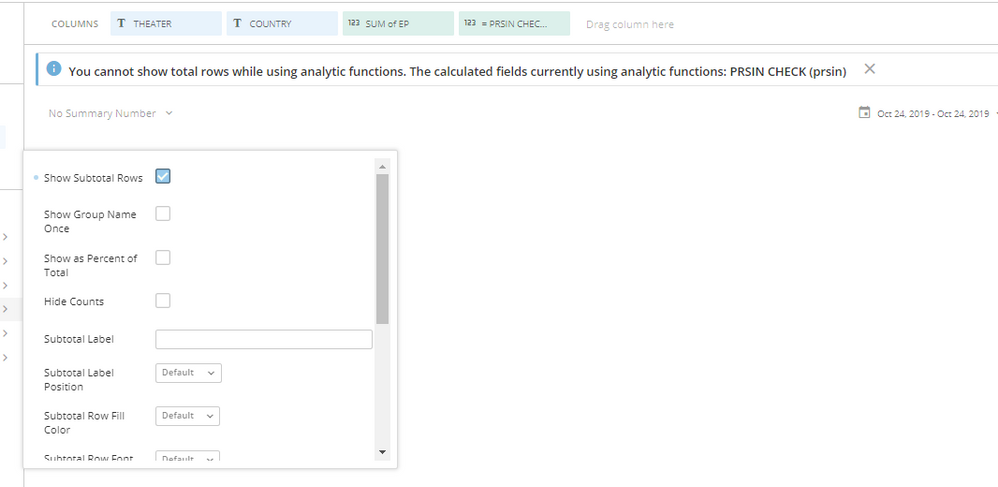# Adding percentage to total but cannot apply a 'Sub Total' in table

Hi,

I have added a beast mode to calculate the % of the total in the table (refer to far left column).Beast mode formula: SUM(`EP`)/SUM(SUM(`EP`)) OVER ()

However, it now gives me an error when I insert a sub total in the table.How can I fix this issue so it can show the sub-total or total row in the table?

Thank you.